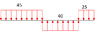# (Weightlifting Machine) Weight stack pin design problem

pin_desinger
Hi and thanks if you decide to go through this post. As part of a school project I'm analysing a weight stack selector pin used in various fitness training equipment. Its material, length and diameter are known.

1. Homework Statement

The pin is placed into a hole equal to its length (from the knob to the tip), which is the hole of the bottom plate of the stack. However the length of the hole is not equal to the length of the whole plate. As the stack is lifted, he pin is being sheared from the plate stack hole and the rod used to lift the stack. It's supposed that we study its behaviour when one is to lift 100kg so applied force to the wire connecting the rod is 1kN. So the total resisting force from the plate stack should be 1 kN as well. Think of this whole setup as a bolt or pin in double shear. What happens in this case however is that the two parts of the plate hole in which the pin is have different length (click here to get a better perspective) and that means the force each part applies to the pin is different. Note that the three applied forces must be considered as three distributed loads. Check attached image for reference. 45 mm is the bigger length of the hole , 40 mm is the length of the rod and 25 mm the smaller length of the hole.

## Homework Equations

I have considered the 1kN to be distributed to each part of the plate in analogy to its length ; e.g. in the 45 mm section we would have 0.7kN and in the 25 one 0.3 kN. So then we can consider the UDLs for all parts respectively.

## The Attempt at a Solution

[/B]
Considering the force analogy to be 70% - 30% and calcuating the shear and bending moment functions, I end up getting values for the bending moment so high that the part fails, while it's actually not supposed to do so. Since I believe my fatigue analysis methods are correct and the material data is valid, there must be something wrong with the force distribution.

Is there a way to find out exactly how much of the 1kN force is distributed to the 45 mm side and how much to the 25 mm one?

Thank you and pardon my terrible English.

#### Attachments

•pin.png
2 KB · Views: 467
Last edited: